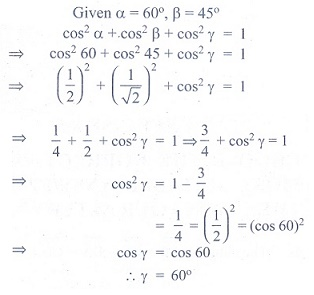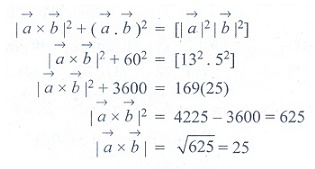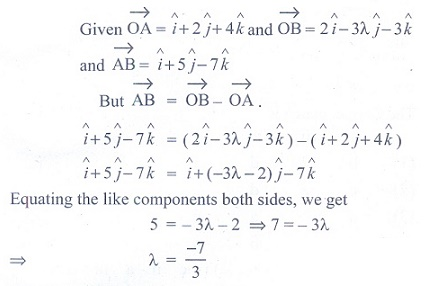Home | | Maths 11th std | Exercise 8.5: Choose the correct answer

# Exercise 8.5: Choose the correct answer

Multiple choice questions with answers / choose the correct answer with answers - Maths Book back 1 mark questions and answers with solution for Exercise Problems - Mathematics : Vector Algebra

Vector Algebra (Mathematics)

## Choose the correct or the most suitable answer from the given four alternatives

(1) The value ofisAns: (3)

Solution(2) Ifare parallel, then the value of m is

(1) 3

(2) 1/3

(3) 6

(4) 1/6

Ans: (3)

Solution(3) The unit vector parallel to the resultant of the vectors iˆ + jˆ − kˆ and iˆ − 2 jˆ + kˆ is

(1) iˆ − jˆ + kˆ  /√5

(2) 2iˆ + jˆ  /√5

(3) 2iˆ − jˆ + kˆ /√5

(4) 2iˆ − jˆ /√5

Ans: (4)

Solution(4) A vectormakes 60° and 45° with the positive direction of the x and y axes respectively. Then the angle betweenand the z-axis is

(1) 45°

(2) 60°

(3) 90°

(4) 30°

Ans: (2)

Solution(5) If= 3iˆ + 2 jˆ + kˆ and the position vector of B is iˆ + 3jˆ − kˆ, then the position vector A is

(1) 4iˆ + 2 jˆ + kˆ

(2) 4iˆ + 5 jˆ

(3) 4iˆ

(4) −4iˆ

Ans: (2)

Solution(6)  A vector makes equal angle with the positive direction of the coordinate axes. Then each angle is equal to

(1) cos-1 (−1/3)

(2) cos−1 (2/3)

(3) cos−1 (1/√3)

(4) cos−1 (2/√3)

Ans: (3)

Solution7) The vectorsare

(1) parallel to each other

(2) unit vectors

(3) mutually perpendicular vectors

(4) coplanar vectors

Ans: (4)

(8) If ABCD is a parallelogram, thenis equal toAns: (4)

Solution(9) One of the diagonals of parallelogram ABCD withandas adjacent sides is+. The other diagonalisAns: (2)

Solution(10) Ifare the position vectors A and B, then which one of the following points whose position vector lies on AB, isAns: (3)

Solution(11) Ifare the position vectors of three collinear points, then which of the following is true?Ans: (2)

Solution(12) If, then the point P whose position vectordivides the line joining the points with position vectorsandin the ratio

(1) 7 : 9 internally

(2) 9 : 7 internally

(3) 9 : 7 externally

(4) 7 : 9 externally

Ans: (1)

Solution(13) If λiˆ + 2λjˆ + 2λkˆ is a unit vector, then the value of λ is

(1) 1/3

(2) 1/4

(3) 1/9

(4) 1/2

Ans: (1)

Solution(14) Two vertices of a triangle have position vectors 3iˆ + 4 ˆj − 4kˆ and 2iˆ + 3 ˆj + 4kˆ. If the position vector of the centroid is iˆ + 2 ˆj + 3kˆ, then the position vector of the third vertex is

(1) −2iˆ − jˆ + 9kˆ

(2) −2iˆ − jˆ − 6kˆ

(3) 2iˆ − jˆ + 6kˆ

(4) −2iˆ + jˆ + 6kˆ

Ans: (1)

Solution(15) If || = 60,|-| = 40 and || = 46, thenis

(1) 42

(2) 12

(3) 22

(4) 32

Ans: (3)

Solution(16) Ifand, having same magnitude and angle between them is 60º and their scalar product is 1/2 then || is

(1) 2

(2) 3

(3) 7

(4) 1

Ans: (4)

Solution(17) The value of θ (0, π/2) for which the vectorsare perpendicular, is equal to

(1) π /3

(2) π/6

(3) π/4

(4) π/2

Ans: (1)

Solution(18)  If ||= 13, ||= 5 and= 60° then |×| is

(1) 15

(2) 35

(3) 45

(4) 25

Ans: (4)

Solution(19) Vectorsandare inclined at an angle θ = 120º. If ||= 1,||= 2 , then [(+ 3) × (3−)]2  is equal to

(1) 225

(2) 275

(3) 325

(4) 300

Ans: (4)

Solution(20) Ifandare two vectors of magnitude 2 and inclined at an angle 60º , then the angle betweenand+is

(1) 30°

(2) 60°

(3) 45°

(4) 90°

Ans: (1)

Solution(21) If the projection of   5iˆ − jˆ − 3kˆ on the vector iˆ + 3jˆ + λkˆ is same as the projection of iˆ + 3 jˆ + λkˆ on 5iˆ − jˆ − 3kˆ, then λ is equal to

(1) ± 4

(2) ± 3

(3) ± 5

(4) ±1

Ans: (3)

Solution(22) If (1, 2, 4) and (2, - 3λ - 3) are the initial and terminal points of the vector iˆ + 5 ˆj − 7kˆ , then the value of λ is equal to

(1) 7/3

(2) − 7/3

(3) − 5/3

(4) 5

Ans: (2)

Solution(23) If the points whose position vectors 10iˆ + 3 ˆj , 12iˆ − 5 ˆj and aiˆ +11ˆj are collinear then a is equal to

(1) 6

(2) 3

(3) 5

(4) 8

Ans: (4)

Solution(24)  If= iˆ + jˆ + kˆ,= 2iˆ + xjˆ + kˆ,= iˆ − jˆ + 4kˆ and⋅ (×) = 70 , then x is equal to

(1) 5

(2) 7

(3) 26

(4) 10

Ans: (3)

Solution(25) If= iˆ + 2 jˆ + 2kˆ, ||= 5 and the angle betweenandis π/6 , then the area of the triangle formed by these two vectors as two sides, is

(1) 7/4

(2) 15/4

(3) 3/4

(4) 17/4

Ans: (2)

SolutionTags : Vector Algebra | Mathematics , 11th Mathematics : UNIT 8 : Vector Algebra I
Study Material, Lecturing Notes, Assignment, Reference, Wiki description explanation, brief detail
11th Mathematics : UNIT 8 : Vector Algebra I : Exercise 8.5: Choose the correct answer | Vector Algebra | Mathematics